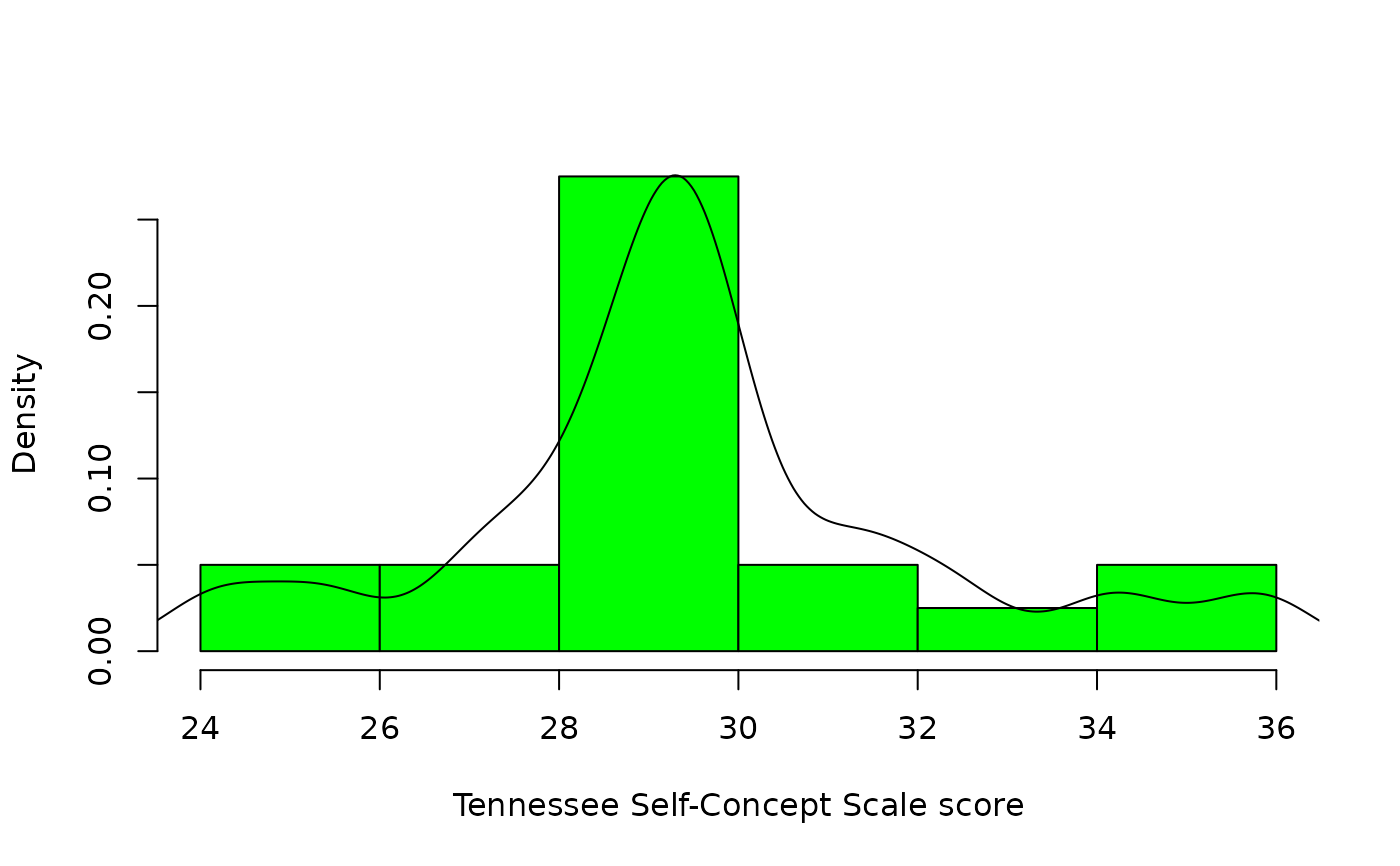Data for Exercise 6.56

Tenness

## Format

A data frame/tibble with 20 observations on one variable

score

Tennessee Self-Concept Scale score

## Examples


hist(Tenness$score, freq= FALSE, main = "", col = "green", xlab = "Tennessee Self-Concept Scale score") lines(density(Tenness$score))if (FALSE) {
library(ggplot2)
ggplot2::ggplot(data = Tenness, aes(x = score, y = ..density..)) +
geom_histogram(binwidth = 2, fill = "purple", color = "black") +
geom_density(color = "red", fill = "pink", alpha = 0.3) +
theme_bw()
}